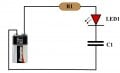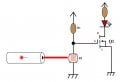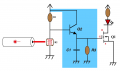# Help : LED Delay OFF

#### Guest3123

Joined Oct 28, 2014
404
Need help with a very simple LED delay off circuit.

I think it's with a resistor and capacitor, but I don't know how the circuit works, or how to calculate how long the led will stay on for.

I've seen this video.. Which shows a 9v battery charging the capacity, and the LED stays on for what seems like a long time.

Sorry about the music, it's not my video.

I've seen stuff like this on YouTube, but don't know the approte search string to search and find more videos like this. Any help would be apreciated.

Something like this...?I'd like keep the LED on for about 3 seconds, then make it go off, either dimming it off, or suddenly make the LED go off.

#### AnalogKid

Joined Aug 1, 2013
9,486
With the circuit you have, the LED will come on immediately when power is connected, then slowly fade to off over time. Once the LED is off, there is no more current flow from the battery (unless it is an extremely poor quality capacitor). If this is the action sequence you want, then what you have is a capacitor being charged up through a resistor, with the LED converting the charging current to light. Initial conditions:

Battery = 9.0 V
LED forward voltage, Vf = 2.0 V.
LED peak current = 20 mA (a typical value for garden-variety LEDs, 0.02 A)
The forward voltage is the voltage drop across the LED when it is "on" and making light.

An R-C timing circuit has an exponential shape. To start off a bit more simply, there is an approximation that assumes a constant charging current and linear voltage change:

EC=IT
E x C = I x T
Voltage times Capacitance = Current times Time
The max voltage across the capacitor is 7 V (9 V battery minus the LED Vf)
C = (I x T) / E
C = (.02 x 3) / 7.0 = 0.0086 = 8600 uF

The resistor is calculated using Ohm's Law.
R=E/I
Resistance = Voltage divided by Current
R = 7 / 0.02 = 350 ohms

Digest this, and we'll move to the exponential equation for a more accurate result.

ak

Last edited:
•Guest3123

#### AnalogKid

Joined Aug 1, 2013
9,486
I just watched the 2nd video. In that, the circuit operation is slightly different in that the LED is on continuously while the power is connected, and then decays after the battery is disconnected. The calculation above applies to the decay part.

ak

#### Guest3123

Joined Oct 28, 2014
404
With the circuit you have, the LED will come on immediately when power is connected, then slowly fade to off over time. Once the LED is off, there is no more current flow from the battery (unless it is an extremely poor quality capacitor). If this is the action sequence you want, then what you have is a capacitor being charged up through a resistor, with the LED converting the charging current to light. Initial conditions:

Battery = 9.0 V
LED forward voltage, Vf = 2.0 V.
LED peak current = 20 mA (a typical value for garden-variety LEDs, 0.02 A)
The forward voltage is the voltage drop across the LED when it is "on" and making light.

An R-C timing circuit has an exponential shape. To start off a bit more simply, there is an approximation that assumes a constant charging current and linear voltage change:

EC=IT
E x C = I x T
Voltage times Capacitance = Current times Time
The max voltage across the capacitor is 7 V (9 V battery minus the LED Vf)
C = (I x T) / E
C = (.02 x 3) / 7.0 = 0.0086 = 8600 uF

The resistor is calculated using Ohm's Law.
R=E/I
Resistance = Voltage divided by Current
R = 7 / 0.02 = 350 ohms

Digest this, and we'll move to the exponential equation for a more accurate result.

ak

It's going to be used for a Laser hitting the photoresistor. I know the circuit and I understand Voltage divider using a n-Channel Mosfet for the laser tripwire.

Kinda like this..So instead of the LED going off immediately, I want it to stay on for a couple seconds.

Ok, now I'm going to look at your reply.. I wanted to just put that there just to show why I wanted to learn about the simple discharging circuit.

I learned last night about the laser tripwire from Afrotechmods.. I understand 100% how to do it, because I learned about the voltage divider, V1 = R1 / (R1 + R2) * Vs, and I also know about the n-Channel mosfet because I've built box mods using them, and I also studied for about a week on them, so I know the circuit, even the math.

LASER ON = 250 / (250 + 2200) = 0.10204081 * 5vdc = 0.510204Vdc
250 + 2200 = 2450Ω

LASER OFF = 4500 / (4500 + 2200) = 0.671641 * 5vdc = 3.3582Vdc
4500 + 2200 = 6700Ω

The n-Channel mosfet would need to have a Vgs(th) of less than 3.35vdc, something like the IRLB8721PbF, but it would also need to be higher than the Min Vgs(th).

•Tonyr1084

#### Guest3123

Joined Oct 28, 2014
404
With the circuit you have, the LED will come on immediately when power is connected, then slowly fade to off over time. Once the LED is off, there is no more current flow from the battery (unless it is an extremely poor quality capacitor). If this is the action sequence you want, then what you have is a capacitor being charged up through a resistor, with the LED converting the charging current to light. Initial conditions:

Battery = 9.0 V
LED forward voltage, Vf = 2.0 V.
LED peak current = 20 mA (a typical value for garden-variety LEDs, 0.02 A)
The forward voltage is the voltage drop across the LED when it is "on" and making light.

An R-C timing circuit has an exponential shape. To start off a bit more simply, there is an approximation that assumes a constant charging current and linear voltage change:

EC=IT
E x C = I x T
Voltage times Capacitance = Current times Time
The max voltage across the capacitor is 7 V (9 V battery minus the LED Vf)
C = (I x T) / E
C = (.02 x 3) / 7.0 = 0.0086 = 8600 uF

The resistor is calculated using Ohm's Law.
R=E/I
Resistance = Voltage divided by Current
R = 7 / 0.02 = 350 ohms

Digest this, and we'll move to the exponential equation for a more accurate result.

ak

And if I wanted to use 5vdc, 2 seconds delay, or decay..

0.02A x 2s = 0.04
Vf = LED's Forward Voltage. (7vdc)
Capacitance = (0.02A x 2s) = 0.04A / 7Vf = 0.005714 or.. 571 Hundred Thousandths of a Farad..?

I'm not too familiar with the decimal formats of Farads.. I'd actually quite new to Farads.. Could you help me..

I tried to look it up on Wikipedia.. but all I see on Wikipedia is..

1 mF (millifarad, one thousandth (10−3) of a farad) = 1000 μF = 1000000 nF
1 μF (microfarad, one millionth (10−6) of a farad) = 1000 nF = 1000000 pF
1 nF (nanofarad, one billionth (10−9) of a farad) = 1000 pF

0.005, So that's 5 Thousandths.
0.0057, that's fifty seven ten thousandths of a farad.
0.00571, and that's.. five hundred and seventy one hundred thousandths of a farad.

Please correct me if I'm wrong, but that's.. 571 uF ?

I know my decimal places, and I know how to do all kinds of stuff with decimal places, but it's the Farads that's confusing. I'm new to them.

So.. if a millifarad being one thousandths of a farad, 0.001F, then It would be something like 5.71mF ?

#### AnalogKid

Joined Aug 1, 2013
9,486
OK... no more baby steps. From 250 to 4500 ohms is not a very big change for an LDR, so less margin for the FET on and off conditions. So, you want a minimum LED on time even if the tripwire break is very short, is that it? If so, then a capacitor across the FET sill rapidly discharge through the FET when the wire is tripped, and slowly recharge through the resistor and LED when the wire is re-established. The LED on time is the variable break time plus the constant recharge time. You probably will have to add another resistor to protect the FET from overcurrent with a large capacitor.

Time constant calculators:
http://hughestech.com/rc_calculator/
https://www.easycalculation.com/engineering/electrical/capacitor-energy-rc-time-constant.php

ak

#### AnalogKid

Joined Aug 1, 2013
9,486
0.00571, and that's.. five hundred and seventy one hundred thousandths of a farad.
Please correct me if I'm wrong, but that's.. 571 uF ?
I know my decimal places, and I know how to do all kinds of stuff with decimal places, but it's the Farads that's confusing. I'm new to them.
So.. if a millifarad being one thousandths of a farad, 0.001F, then It would be something like 5.71mF ?
You dropped a decimal place, then got it back at the end. 5.71 mF is correct, but more commonly described as 5710 uF. millifarads is almost never used.

ak

Last edited:

#### Guest3123

Joined Oct 28, 2014
404
OK... no more baby steps. From 250 to 4500 ohms is not a very big change for an LDR, so less margin for the FET on and off conditions. So, you want a minimum LED on time even if the tripwire break is very short, is that it? If so, then a capacitor across the FET sill rapidly discharge through the FET when the wire is tripped, and slowly recharge through the resistor and LED when the wire is re-established. The LED on time is the variable break time plus the constant recharge time. You probably will have to add another resistor to protect the FET from overcurrent with a large capacitor.

Time constant calculators:
http://hughestech.com/rc_calculator/
https://www.easycalculation.com/engineering/electrical/capacitor-energy-rc-time-constant.php

ak

It's good to have baby steps.. I'm a slow learner. But basically, I know how to find the Capacitor now. Thanks to you. As long as it charges the capacitor FAST, and discharges it in a timely manner, I'm good. Yes, I want the LED to light for about 2 - 3 seconds. If the tripwire get's tripped, the led might blink twice, or if the trip wire is on a step, then the led might stay lit for even longer, depending on how fast the person is coming up the stairs. etc.

But if it were flat ground, and it wasn't a human I'm trying to sense, and it's a rabbit, or whatever, then I'd need an R-C Circuit, and I'd want the cap to charge quickly, and discharge in a timely manner. I think I've got it.

As for protecting the FET, I'd sure I will not have to protect it, as it's good for about 6 Amps. So.. No more resistors.

Last edited:

#### Guest3123

Joined Oct 28, 2014
404
You dropped a decimal place, then got it back at the end. 5.7f mF is correct, but more commonly described as 5700 uF. millifarads is almost never used.

ak
I understand. Because there's 1000 uF in 1mF. So just use uF instead, and it becomes 5710uF, which I'm not sure Mouser Electronics sells them, but I'll take a look later, even though I probably wont use that circuit, but the 3s circuit instead.

I'm also aware that I'll need to recalculate the resistor value, as well as the R-C Circuit because I'm using a different voltage, so everything changes..

#### dannyf

Joined Sep 13, 2015
2,197
"The n-Channel mosfet would need to have a Vgs(th) of less than 3.35vdc,"

I think you will find it hard to hit that consistently.

A better approach is to use a comparator to sharpen the on and off character of your circuit.

A comparator can be a standard comparator / opamp, a voltage ref like tl431 or a logic gate....

#### Tonyr1084

Joined Sep 24, 2015
6,453
OK, The blue highlighted circuitry will do what you ask. When the laser beam is interrupted the resistance of R2 goes way up. That turns Q2 on, charging C1 and powering the Gate on Q1, thus lighting the LED. When the beam is restored Q2 drops out and C1 MUST be drained through R3. Failure to drain C1 will hold Q1 active for months. The size of the capacitor and the value of the resistor will determine the decay rate.#### Guest3123

Joined Oct 28, 2014
404
OK, The blue highlighted circuitry will do what you ask. When the laser beam is interrupted the resistance of R2 goes way up. That turns Q2 on, charging C1 and powering the Gate on Q1, thus lighting the LED. When the beam is restored Q2 drops out and C1 MUST be drained through R3. Failure to drain C1 will hold Q1 active for months. The size of the capacitor and the value of the resistor will determine the decay rate.

View attachment 103574
Thanks Tony.

#### dannyf

Joined Sep 13, 2015
2,197
'The blue highlighted circuitry will do what you ask.'

Unlikely to work as is. The capacitor is charged up when the light isn't on and the more Si when the light us on.

Use a PNP instead or swap the ldr with r1.

#### AnalogKid

Joined Aug 1, 2013
9,486
Balancing resistances might be tricky, but it should work as is.

When the beam is broken, Q2 emitter goes up and turns on Q1 and charges C1. I wouldn't say "on" since that implies a switching action, and Q1 is an emitter follower. When the beam returns, Q2 is cut off and C1 holds Q1 on until is is discharged by R3. The LED will turn off gradually, but faster than if it were powered by C1 directly.

If you change Q2 to a PNP acting as a saturated switch, you must (not "or") swap R1 and R2 to have the same circuit function.

ak

#### dannyf

Joined Sep 13, 2015
2,197
'The blue highlighted circuitry will do what you ask.'

There are some other issues that you may have to solve for the circuit to be usable, aside from the placement of LDR/R1 or the NPN vs. PNP issue I posted earlier.

For example, what controls the charge-up current into the capacitor?

Also, as is, the drop off of the current going through the LED will be quite sharp -> the led will appear to go dim suddenly, not gradually.

#### dannyf

Joined Sep 13, 2015
2,197
Kinda like this..
That circuit can be easily made to work as you desired by using a p-ch mosfet: if n-ch mosfet is used (as is), the circuit turns on the led gradually after the laser has been removed.

To make that circuit work as you desired, you will need to swap R1/LDR, and put a rc network from the (n-ch) mosfet's gate to ground. You may have to experiment with the values for desired bahaviors but it can be made to work - the use of a transistor to separate the sensing of the laser + charging of the capacitor greatly expands your choice of components.

#### Marcus2012

Joined Feb 22, 2015
424
Hey all, If no one has mentioned it already can I suggest considering a Photodiode instead of an LDR? It has a faster photointeruption properties, a better Half angle (so it will pick up less undirected ambient light), and the SFH213 (which I used for a fast acting laser trip) has 80% spectral sensitivity at 650nm.

#### dannyf

Joined Sep 13, 2015
2,197
This is what I'd like to learn about..
What kind of language was she speaking? kind English and kind something else.

#### dannyf

Joined Sep 13, 2015
2,197
can I suggest considering a Photodiode instead of an LDR?
You pretty much have to use an amplifier for those things as they generate very little current -> high resistance source. LDR is the right choice here.